Related Articles

# Class 9 RD Sharma Solutions – Chapter 8 Introduction to Lines and Angles- Exercise 8.4 | Set 1

• Last Updated : 28 Apr, 2021

### Question 1: In figure, AB, CD and ∠1 and ∠2 are in the ratio 3 : 2. Determine all angles from 1 to 8.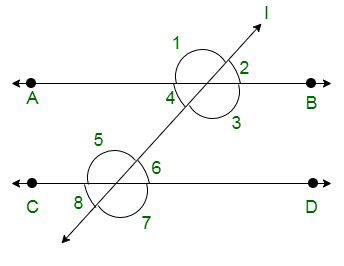Solution:

Assume,

∠1 = 3x and ∠2 = 2x

From the following figure: ∠1 and ∠2 are a linear pair of angles

Thus,

∠1 + ∠2 = 180°

3x + 2x = 180°

5x = 180°

x =x = 36°

Hence,

∠1 = 3x = 108°

and

∠2 = 2x = 72°

We know that:

(Vertically opposite angles are equal)

Here the pairs of vertically opposite angles are:

(∠1 = ∠3);

(∠2 = ∠4);

(∠5, ∠7)

and

(∠6 = ∠8)

∠1 = ∠3 = 108°

∠2 = ∠4 = 72°

∠5 = ∠7

∠6 = ∠8

Now as it is known

(if a transversal intersects any parallel lines, then the corresponding angles are equal)

∠1 = ∠5 = ∠7 = 108°

∠2 = ∠6 = ∠8 = 72°

Hence,

∠1 = 108°, ∠2 = 72°, ∠3 = 108°, ∠4 = 72°, ∠5 = 108°, ∠6 = 72°, ∠7 = 108° and ∠8 = 72°

### Question 2: In figure, I, m, and n are parallel lines intersected by transversal p at X, Y and Z respectively. Find ∠1, ∠2, and ∠3.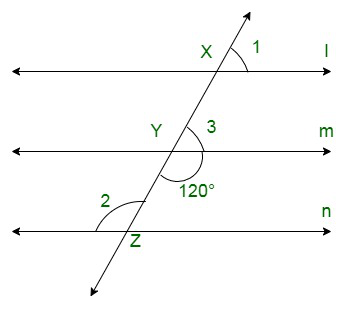Solution:

Here as given in the figure

∠Y = 120° {Vertical opposite angles]}

∠3 + ∠Y = 180° {Linear pair angles}

∠3= 180 – 120

⇒ ∠3= 60°

As we can see the line ‘l’ is parallel to line ‘m’,

∠1 = ∠3 {Corresponding angles}

∠1 = 60°

Now, line ‘m’ is parallel to line ‘n’,

∠2 = ∠Y {Alternate interior angles}

∠2 = 120°

∠1 = 60°,

∠2 = 120°

and

∠3 = 60°.

### Question 3: In figure, AB || CD || EF and GH || KL. Find ∠HKL.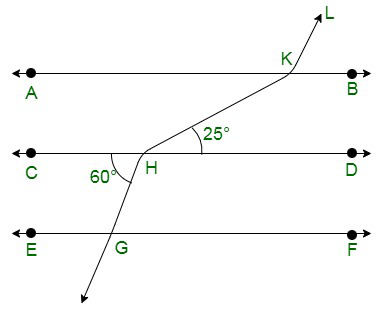Solution:

Construct: Extend LK to meet line GF at point P.

As shown below.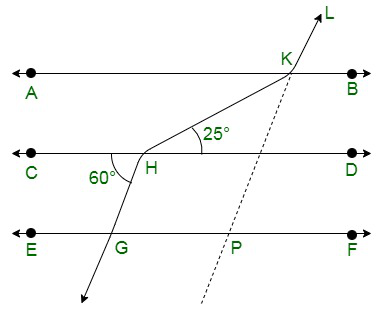Here as from the figure,

CD || GF,

∠CHG =∠HGP = 60° {alternate angles}

∠HGP =∠KPF = 60° {Corresponding angles of parallel lines}

Thus,

∠KPG =180° – 60°

⇒∠KPG = 120°

∠GPK = ∠AKL= 120° {Corresponding angles of parallel lines}

∠AKH = ∠KHD = 25° {alternate angles of parallel lines}

Thus,

∠HKL = ∠AKH + ∠AKL

⇒25 + 120

⇒∠HKL = 145°

### Question 4: In figure, show that AB || EF.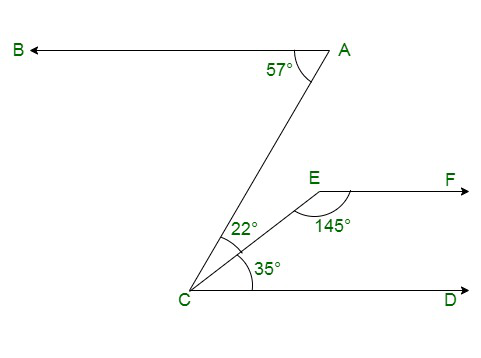Solution:

Construct: Produce EF to intersect AC at point N.

As it is seen in the figure: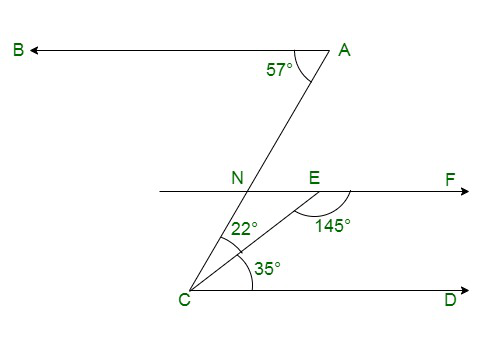∠BAC = 57°

and

∠ACD = 22°+35° = 57°

{Alternative angles of parallel lines are equal}

BA || EF …..(i)

We know that,

Sum of Co-interior angles of parallel lines is 180°

EF || CD

∠DCE + ∠CEF = 35 + 145 = 180° …(ii)

From (i) and (ii)

AB || EF {Lines parallel to the same line are parallel to each other}

Hence Proved.

### Question 5: In figure, if AB || CD and CD || EF, find ∠ACE.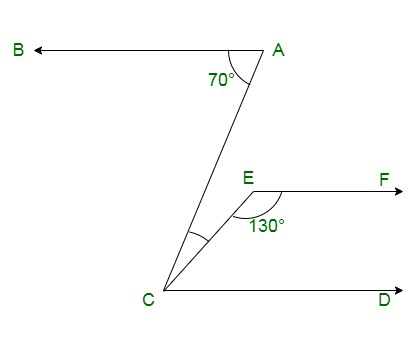Solution:

Given:

CD || EF

∠ FEC + ∠ECD = 180° {Sum of co-interior angles is supplementary to each other}

∠ECD = 180° – 130° = 50°

Now, BA || CD

∠BAC = ∠ACD = 70° {Alternative angles of parallel lines are equal}

Thus,

∠ACE + ∠ECD =70°

∠ACE = 70° – 50°

∠ACE = 20°

### Question 6: In figure, PQ || AB and PR || BC. If ∠QPR = 102°, determine ∠ABC. Give reasons.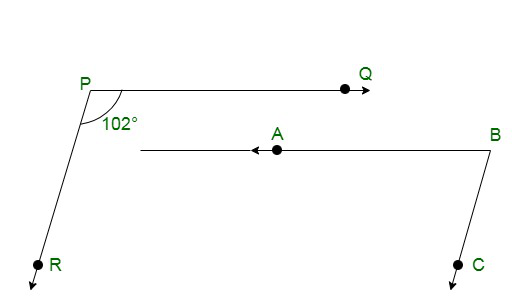Solution:

Construct:

Extend line AB to meet line PR at point G.

As shown below;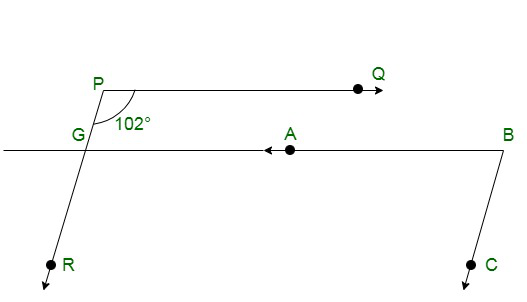Given:

PQ || AB,

∠QPR = ∠BGR =102° {Corresponding angles of parallel lines}

And

PR || BC,

∠RGB+ ∠CBG =180° {Corresponding angles are supplementary}

∠CBG = 180° – 102° = 78°

Thus,

∠CBG = ∠ABC

⇒ ∠ABC = 78°

### Question 7: In figure, state which lines are parallel and why?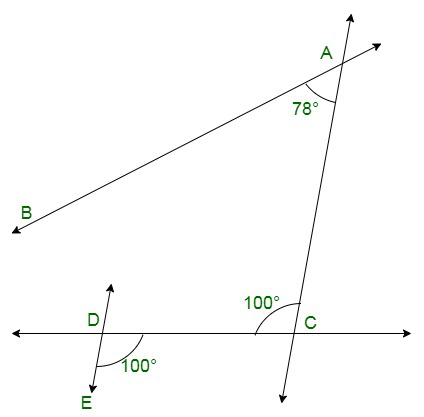Solution:

As we know that,

If a transversal intersects two lines such that a pair of alternate interior angles are equal, then the two lines are parallel

As we can see from the figure:

⇒ ∠EDC = ∠DCA = 100°

Lines DE and AC are intersected by a transversal DC such that the pair of alternate angles are equal.

Hence,

DE || AC

### Question 8: In figure, if l||m, n || p and ∠1 = 85°, find ∠2.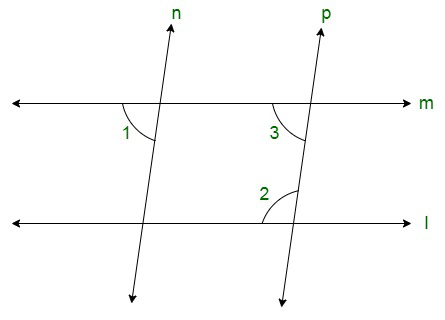Solution:

Given:

∠1 = 85°

We know that,

When a line cuts the parallel lines, the pair of alternate interior angles are equal.

⇒ ∠1 = ∠3 = 85°

Thus again, co-interior angles are supplementary,

Therefore

∠2 + ∠3 = 180°

∠2 + 55° =180°

∠2 = 180° – 85°

∠2 = 95°

### Question 9: If two straight lines are perpendicular to the same line, prove that they are parallel to each other.

Solution:

Assume lines ‘l’ and ‘m’ are perpendicular to ‘n’,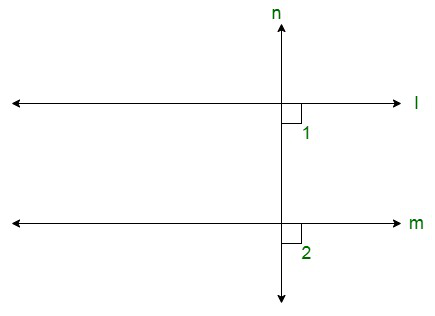Thus

∠1= ∠2=90°

Therefore,

The lines ‘l’ and ‘m’ are cut by a transversal line i.e. ‘n’

The corresponding angles are equal, so it can be seen that,

Line ‘l’ is parallel to line ‘m’.

### Question 10: Prove that if the two arms of an angle are perpendicular to the two arms of another angle, then the angles are either equal or supplementary.

Solution:

Consider the angles be ∠ACB and ∠ABD

Let AC perpendicular to AB, and

CD is perpendicular to BD.

To Prove:

∠ACD = ∠ABD

∠ACD + ∠ABD =180°

Proof :

∠A+ ∠C+ ∠D+ ∠B = 360° {Sum of angles of quadrilateral is 360°}

180° + ∠C + ∠B = 360°

∠C + ∠B = 360° –180°

Thus,

∠ACD + ∠ABD = 180°

And

∠ABD = ∠ACD = 90°

Therefore, angles are equal as well as supplementary.

Attention reader! All those who say programming isn’t for kids, just haven’t met the right mentors yet. Join the  Demo Class for First Step to Coding Coursespecifically designed for students of class 8 to 12.

The students will get to learn more about the world of programming in these free classes which will definitely help them in making a wise career choice in the future.

My Personal Notes arrow_drop_up